#### Mechanics, Strength of Materials and Theory of Structures

SHEAR FORCE & BENDING MOMENT
PURE BENDING
SOLVED EXAMPLES BASED ON SHEAR FORCE AND BENDING MOMENT DIAGRAMS
FLEXURAL STRESS

## PURE BENDING

PURE BENDING

18.1 Introduction

Pure bending refers to flexure of a beam under a constant bending moment. Therefore, pure bending occurs only in regions of a beam where the shear force is zero. In contrast, non uniform bending refers to flexure in the presence of shear forces, which means that the bending moment changes as we move along the axis of the beam. An example of pure bending would be a beam with two couples, one on each end acting in opposite directions.

18.2 Assumption in the Theory of Simple Bending

The following assumption is made in the theory of simple bending:

1. The material of the beam is perfectly homogeneous (i.e., of the same kind throughout) and isotropic (i.e., of equal elastic properties in all directions).
2. The beam materials are stressed within its elastic limit and, thus, obey Hooks law.
3. The transverse sections, which were plane before bending, remain plane after bending also.
4. Each layer of the beam is free to expand or contract, independently, of the layer, above or below it.
5. The value of E(Young modulus of elasticity) is the same in tension and compression

18.3 Theory of Simple Bending

Consider a small length dx of a simply supported beam subjected to a bending moment M. Now consider two section AB and CD, which are normal to the axis of the beam RS. Due to the action of the bending moment, the beam as a whole will bend. Since we are considering a small length of dx of the beam, therefore, the curvature of the beam, in this length, is taken to be circular. A little consideration will show, that all the layer of the beam, which were originally of the same length doing not remain of the same length any more. The top layer of the beam AC has suffered compression, and reduced to A1C1. As we proceed towards the lower layer of the beam, we find that the layers have, no doubt, suffered compression, but to a lesser degree; until we come across the layer RS, which has suffered no change in its length, through bent into R1S1. If we further proceed towards the lower layers are stretched; the amount of extension increases as we proceed lower, until we come across the lowermost layer BD which has been stretched to B1D1.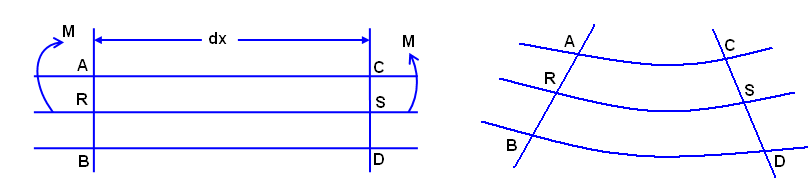Fig. 18.1 simple bending

Now we see that the layers above RS have been compressed and that bellow have stretched. The amount, by which a layer is compressed or stretched, depends upon the position of the layer with reference to RS. This layer RS, which is neither compressed nor stretched, is known as neutral plane or neutral layer. The theory of bending is called theory of simple bending.

18.4 Bending Stress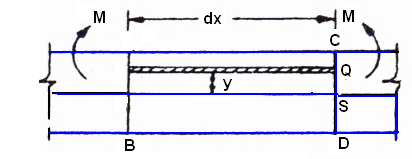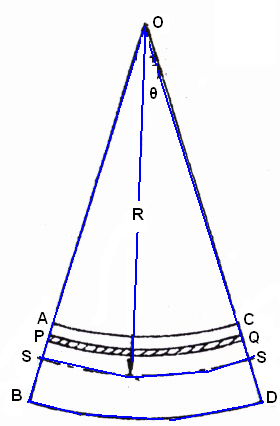(A) Before Bending B) After Bending

Fig. 18.2 Bending stress

Consider a small length dx of a beam subjected to a bending moment. As a result of this moment, let this small length of beam bend into an arc of a circle with O a center.

Let M= Moment acting at the beam,

θ = angle subtended at the center by the arc, and

R= Radius of curvature of the beam

Now consider a layer PQ at a distance y from RS the neutral axis of the beam. Let this layer be compressed to P1Q1 after bending.

Decrease in length of this layer

δl = PQ - P1Q1

Strain e = (δl / Original length) = (PQ - P1Q1) / (PQ)

Now from the geometry of the curved beam, we find that the two section OP1Q1 and OR1S1 are similar

(P1Q1) / (R1S1) = (R - y) / R

Or

1 - (P1Q1) / (R1S1) = 1 - (R - y) / R

Or

(R1S1) - (P1Q1) / (PQ) = ( y / R)

(PQ) - (P1Q1) / (PQ) = ( y / R) ( PQ = R1S1 = RS = Neutral axis)

e = (y / R) ( e =(PQ) - (P1Q1) / (PQ) )

It is thus obvious, that the stain of a layer is proportional to its distance from the neutral axis.

We also know that the stress,

f = Strain X Elasticity = e.E

= (y / R) x E = y x (E / R) ( e = y / R)

Since E and R are constants in this expression, therefore the stress at any point is directly proportional to y, i.e., the distance of the point from the neutral axis.

The above expression may also be written as:

f / y = E / R ----------(1)

18.5 Position if Neutral Axis

The line of intersection of the neutral layer, with any normal cross-section of a beam, is known as neutral axis of the section. We have that on one side of the neutral axis there are compressive stresses, whereas on the other there are tensile stresses. At the neutral axis, there is no stress of any kind.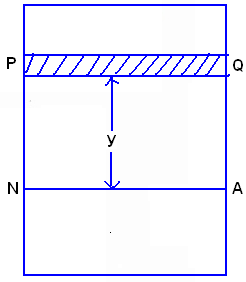Fig. 18.3 Position of neutral axis

18.6 Moment of Resistance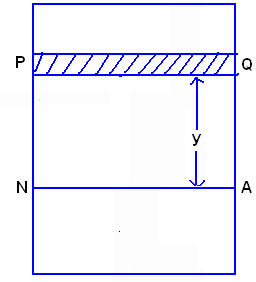Fig. 18.4 Moment of resistance

We have already known that on one side of the neutral axis there are compressive stresses, and on the other there are tensile stresses. These stresses from a couple, whose moment must be equal to the external moment M. The moment of this couple, which resist the external bending moment, is known as moment of resistance. Consider a section of the beam.

Let NA be the neutral axis of the section. Now consider a small layer PQ of the beam section at a distance y from the neutral axis.18.7 Section Modulus

We have

M / I=б/y=E / R

M / I= б / y

б or ƒs =M / I*y

б max =M /I /ymax where Z = I/ymax

= M / Z Z=section modulus.

18.8 Examples of Section Modulus for Different Sections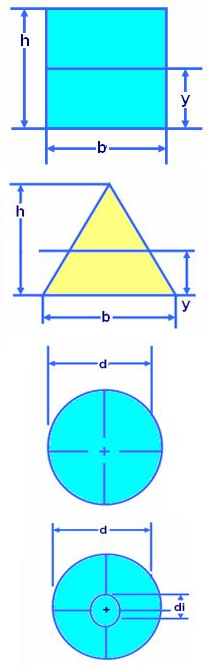Fig. 18.5 Examples of section modulus for different sections

I = Moment of inertia, m^4 ,m4 Z = Section modulus, m^3 , m3

y = Centroidal distance, m

I = b * h^3 / 12 = 1/12 b.h3

Z = b * h^2 / 6 = 1/6 b.h2

y = h / 2

I = b * h^3 / 36 = b.h3/36

Z = b * h^2 / 12 = b.h2/12

y = h / 3

I = ∏d/ 64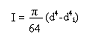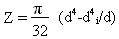y = d / 2